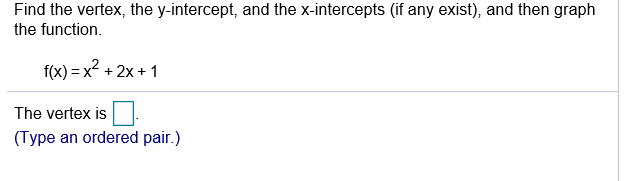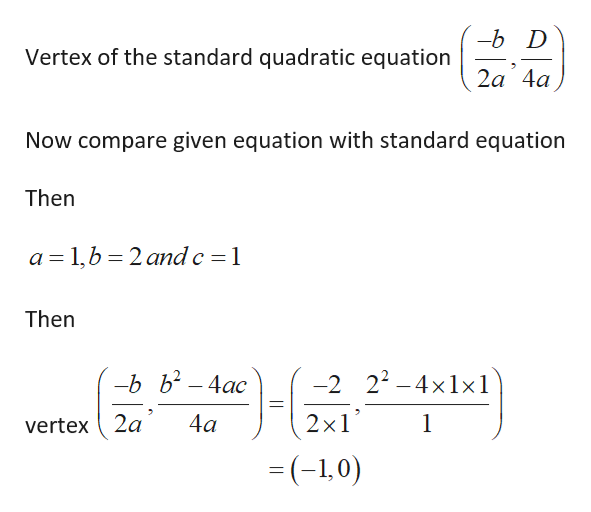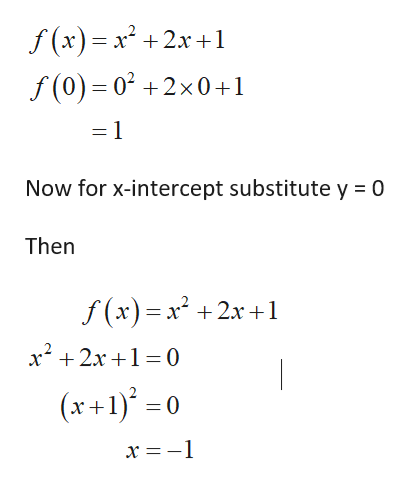# Find the vertex, the y-intercept, and the x-intercepts (if any exist), and then graphthe function.f(x) = x2 + 2x + 1The vertex is(Type an ordered pair.)

Question
4 viewshelp_outlineImage TranscriptioncloseFind the vertex, the y-intercept, and the x-intercepts (if any exist), and then graph the function. f(x) = x2 + 2x + 1 The vertex is (Type an ordered pair.) fullscreen
check_circle

Step 1

Consider the given equation

Step 2

So given equation is quadratic equation

Thenhelp_outlineImage Transcriptionclose--b D Vertex of the standard quadratic equation 2a 4a Now compare given equation with standard equation Then a = 1,b = 2 and c = 1 Then -b b² – 4ac -2 22 – 4×1×1 4a 2x1 vertex ( 2a = (-1,0) fullscreen
Step 3

So for y intercept substitu...help_outlineImage Transcriptionclosef (x)= x² +2x+1 f (0) = 0² +2x0+1 Now for x-intercept substitute y = 0 Then f (x)= x² +2x+1 x? + 2x+1= 0 (x+1)° = 0 x = -1 fullscreen

### Want to see the full answer?

See Solution

#### Want to see this answer and more?

Solutions are written by subject experts who are available 24/7. Questions are typically answered within 1 hour.*

See Solution
*Response times may vary by subject and question.
Tagged in

### Equations and In-equations